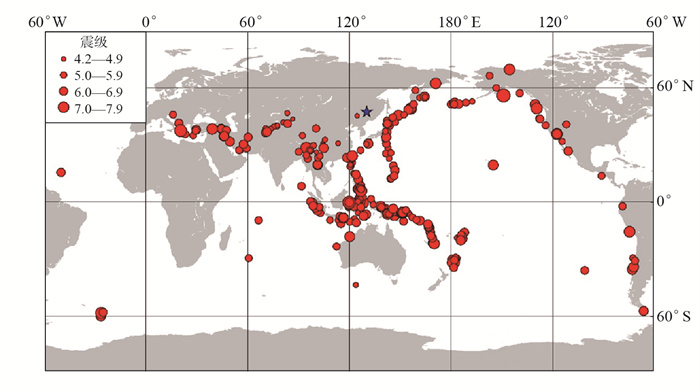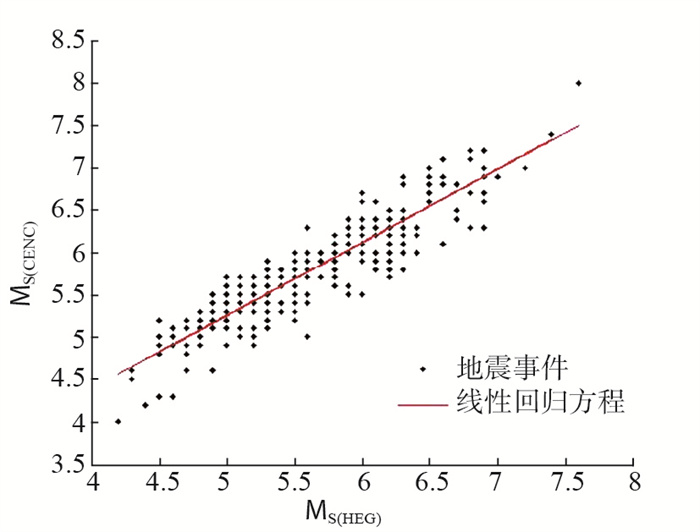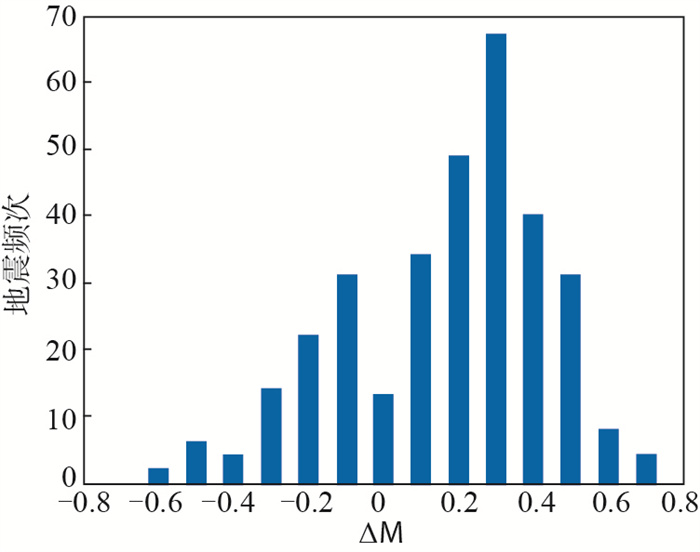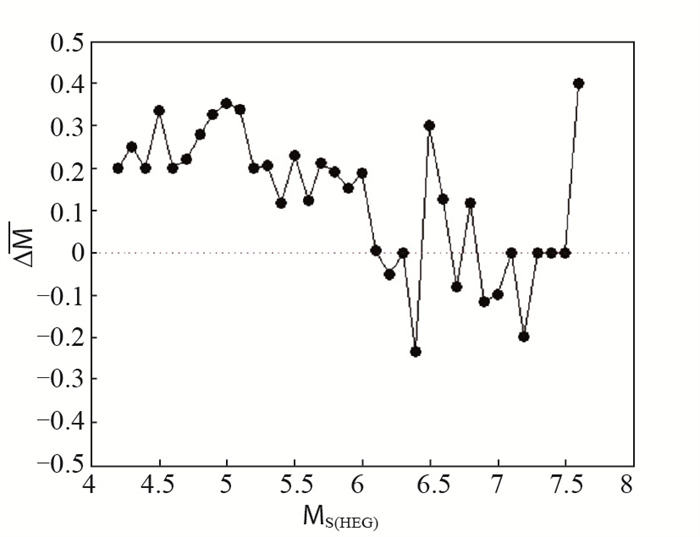﻿ 鹤岗地震台面波震级偏差分析文章快速检索
 地震地磁观测与研究2021, Vol. 42Issue (3): 34-38  DOI: 10.3969/j.issn.1003-3246.2021.03.0040

### 引用本文LI Dawei, ZHANG Hao, JIANG Bo. Analysis of surface wave magnitude deviation at Hegang Seismic Station[J]. Seismological and Geomagnetic Observation and Research, 2021, 42(3): 34-38. DOI: 10.3969/j.issn.1003-3246.2021.03.004.### 文章历史

Analysis of surface wave magnitude deviation at Hegang Seismic Station
LI Dawei, ZHANG Hao , JIANG Bo
Hegang Seismic Station, Heilongjiang Province 154100, China
Abstract: After the publishing of the latest national standard for earthquake magnitude "General rule for earthquake magnitude" (GB17740—2017), the surface wave magnitudes for teleseismics measured by Hegang Seismic Station (HEG) from January 2018 to March 2020 is summarized and compared with the magnitudes published by China Earthquake Networks Center (CENC). The surface wave magnitude deviations between results from HEG and CENC were calculated. By the statistical method, the relationship between magnitude deviation and magnitude value, epicentral distance, and back azimuth was analyzed. The results show that the surface wave magnitude measured by Hegang Seismic Station is higher than that published by China Earthquake Networks Center, and the magnitude deviation is positively correlated with the epicentral distance. Magnitude deviation is smaller for earthquakes to the northwest of Hegang Seismic Station and larger for others.
Key words: surface wave magnitude    magnitude deviation    epicentral distance    back azimuth
0 引言

1 资料选取图 1 本研究所采用地震事件震中分布 Fig.1 Epicenter distribution map of earthquakes used in this study
2 震级测定原理与震级偏差统计 2.1 震级测定

 ${M_{\rm{S}}} = \lg (\frac{A}{T}) + \sigma (\Delta) + C$ (1)

2.2 震级偏差统计

 $\begin{array}{*{20}{l}} {{M_{{\rm{S}}\left({{\rm{CENC}}} \right)}} = {\rm{ }}0.9316{M_{{\rm{S}}\left({{\rm{HEG}}} \right)}} + {\rm{ }}0.8643} \end{array}\;\;\;{\sigma ^2} = 0.2633$ (2)图 2 面波震级线性回归结果 Fig.2 Results of linear regression of surface wave magnitude
 $\Delta M = {M_{{\rm{S}}\left( {{\rm{HEG}}} \right)}} - {M_{{\rm{S}}\left( {{\rm{CENC}}} \right)}}$ (3)表 1 震级偏差统计 Table 1 Statistics of magnitude deviation图 3 震级偏差直方图 Fig.3 Histogram of magnitude deviation

 $\Delta \bar M = \sum\limits_{}^{} {_{i = 1}^n} \Delta {M_i}/N$ (4)
 $\delta = \sqrt {\sum\limits_{}^{} {_{i = 1}^n} {{(\Delta \bar M - \Delta {M_i})}^2}/(n - 1)}$ (5)

3 震级偏差分析 3.1 震级大小与震级偏差的对应关系图 4 MS(HEG)震级大小与震级偏差对应关系 Fig.4 Relationship between magnitude and magnitude deviation
3.2 震级偏差与震中距的关系表 2 震级偏差与震中距的对应关系 Table 2 The relation between magnitude deviation and epicentral distance
3.3 震级偏差与台站反方位角的关系表 3 震级偏差与反方位角的对应关系 Table 3 Relationship between magnitude deviation and back azimuth
4 结论

 何晓群, 刘文 卿.. 应用回归分析[M]. 北京: 中国人民大学出版社, 2001. 何奕成, 秦磊, 立凯, 等. 连云港地震台面波震级偏差分析[J]. 地震地磁观测与研究, 2016, 37(6): 38-42. 刘红桂. 不同构造区固有吸收衰减和散射衰减相对强弱的对比研究[J]. 国际地震动态, 2012(1): 31-32. 刘瑞丰, 陈运泰, 许绍燮, 等. GB17740-2017地震震级的规定[S]. 北京: 中国标准出版社, 2017. 刘瑞丰, 陈运泰, 薛峰, 等. 如何正确使用新的震级国家标准[J]. 地震地磁观测与研究, 2018, 39(4): 1-11. 田文德, 胡俊明. 成都台数字地震仪测定震级偏差分析与校正[J]. 四川地震, 2012(3): 20-23. 中国地震局. 地震参数[M]. 北京: 地震出版社, 2003.